# Java Conditional or Relational Operators

## Description

If you need to change the execution of the program based on a certain condition you can use “if” statements. The relational operators determine the relationship that one operand has to the other. Specifically, they determine equality condition. Java provides six relational operators, which are listed in below table.

Operator Description Example (a=10, b=15) Result
== Equality operator a==b false
!= Not Equal to operator a!=b true
> Greater than a>b false
< Less than a<b true
>= Greater than or equal to a>=b false
<= Less than or equal to a<=b true

The outcome of these operations is a boolean value. The relational operators are most frequently used in the expressions that control the if statement and the various loop statements. Any type in Java, including integers, floating-point numbers, characters, and booleans can be compared using the equality test, ==, and the inequality test, !=. Notice that in Java equality is denoted with two equal signs (“==”), not one (“=”).

In Java, the simplest statement you can use to make a decision is the if statement. Its simplest form is shown here:

```if(condition) statement;
or
if (condition) statement1;
else statement2;
```

Here, the condition is a Boolean expression. If the condition is true, then the statement is executed. If the condition is false, then the statement is bypassed. For example, suppose you have declared an integer variable named someVariable, and you want to print a message when the value of someVariable is 10. Flow chart and java code of the operation looks like below,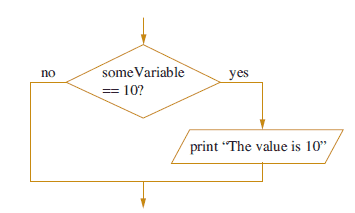``````if (someVariable ==10){
System.out.println("The Value is 10”);
}
```
```

We can have different flavors of if statements.

Nested if blocks:

A nested if is an if statement that is the target of another if or else. In other terms, we can consider one or multiple if statement within one if block to check various condition. For example, we have two variables and want to check particular condition for both we can use nested if blocks.

Java Code:Go to the editor

``````public class NestedIfDemo {
public static void main(String[] args) {
int a =10;
int b =5;
if(a==10){
if(b==5){
System.out.println("Inside Nested Loop");
}
}
}
}
```
```

We might get a situation where we need to check value multiple times to find exact matching condition. Below program explains the same thing. Let’s see we have a requirement to check if the variable value is less than 100, equal to 100 or more than 100. Below code explains the same logic using if-else-if ladder.

Java Code:Go to the editor

``````public class IfelseLadderDemo {
public static void main(String[] args) {
int a =120;
if(a< 100){
System.out.println("Variable is less than 100");
}
else if(a==100)
{
System.out.println("Variable is equal to 100");
}
else if (a>100)
{
System.out.println("Variable is greater than 100");
}
}
}
```
```

Output: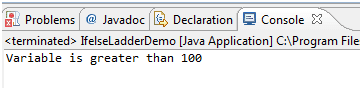Let’s take an example to understand above logical operators and conditional statements. We have a requirement to print result in a grade based on marks entered by the user. We are taking input from an user at runtime and evaluate grades. This program also validates input and prints appropriate message if the input is a negative or alphabetic entry.

Java Code: Go to the editor

``````import java.util.Scanner;
public static void main(String[] args) {
int marks=0	;
try{
//Scanner class is wrapper class of System.in object
Scanner inputDevice = new Scanner(System.in);
marks = inputDevice.nextInt();
//Checking input validity and grade based on input value
if(marks< 0){
System.out.println("Marks can not be negative: Your entry= "+ marks );
}else if(marks==0){
System.out.println("You got Zero Marks: Go to ZOO");
}else if (marks>100){
System.out.println("Marks can not be more than 100: Your entry= "+ marks );
}else if (marks>0 & marks< 35){
System.out.println("You need to work hard: You failed this time with marks ="+ marks);
}else if (marks>=35 & marks < 50){
}else if (marks>=50 & marks < 60){
System.out.println("You can improve performance, you got C+ grade");
}else if (marks>=60 & marks < 70){
}else if (marks>=70 & marks < 80){
}else if (marks>=80 & marks < 90){
}else if (marks>=90){
}
}catch (Exception e){
//This is to handle non-numeric values
System.out.println("Invalid entry for marks:" );
}
}
}
```
```

Outputs based on users input :Short-Circuit Logical Operators

Java provides two interesting Boolean operators not found in many other computer languages. These are secondary versions of the Boolean AND and OR operators and are known as short-circuit logical operators. Two short-circuit logical operators are as follows,

• && short-circuit AND
• || short-circuit OR

They are used to link little boolean expressions together to form bigger boolean expressions. The && and || operators evaluate only boolean values. For an AND (&&) expression to be true, both operands must be true. For example, The below statement evaluates to true because of both operand one (2 < 3) and operand two (3 < 4) evaluate to true.

```if ((2 < 3) && (3 < 4)) { }
```

The short-circuit feature of the && operator is so named because it doesn't waste its time on pointless evaluations. A short-circuit && evaluates the left side of the operation first (operand one), and if it resolves to false, the && operator doesn't bother looking at the right side of the expression (operand two) since the && operator already knows that the complete expression can't possibly be true.

The || operator is similar to the && operator, except that it evaluates to true if EITHER of the operands is true. If the first operand in an OR operation is true, the result will be true, so the short-circuit || doesn't waste time looking at the right side of the equation. If the first operand is false, however, the short-circuit || has to evaluate the second operand to see if the result of the OR operation will be true or false.

Java Code:  Go to the editor

``````public class ShortCircuitOpDemo {
public static void main(String[] args) {
float number1 = 120.345f;
float number2 = 345.21f;
float number3 = 234.21f;
if(number1 < number2 && number1< number3){
System.out.println("Smallest Number is number1");
}
if(number3 >number2 || number3 > number1){
System.out.println("number3 is not smallest");
}
}
}
```
```

Output: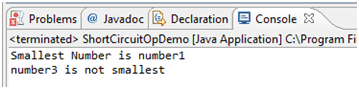Ternary Operator (or ? Operator or Conditional Operator)

The conditional operator is a ternary operator (it has three operands) and is used to evaluate boolean expressions, much like an if statement except instead of executing a block of code if the test is true, a conditional operator will assign a value to a variable. A conditional operator starts with a boolean operation, followed by two possible values for the variable to the left of the assignment (=) operator. The first value (the one to the left of the colon) is assigned if the conditional (boolean) test is true, and the second value is assigned if the conditional test is false. In below example, if variable a is less than b then tje variable x value would be 50 else x =60.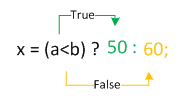below example, we are deciding the status based on user input if pass or failed.

Java Code: Go to the editor

``````import java.util.Scanner;
public class TernaryOpDemo {
public static void main(String[] args) {
//Checking if marks greater than 35 or not
String status;
int marks;
Scanner inputDevice = new Scanner(System.in);
marks = inputDevice.nextInt();
status= marks>=35 ?"You are Passed":"You are failed";
System.out.println("Status= " + status);
}
}
```
```

Outputs based on users input :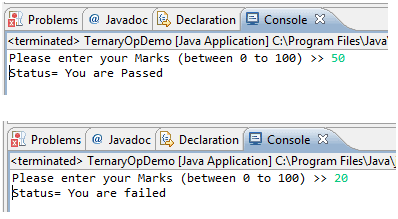Summary

• Java provides six conditional operators == (equality), > (greater than), < (less than), >=(greater or equal), <= (less or equal), != (not equal)
• The relational operators are most frequently used to control the flow of program.
• Short-Circuit logical operators are && and ||
• The ternary operator is one which is similar to if else block but which is used to assign value based on condition.

Java Code Editor:

Previous: Arithmetic Operator
Next: Logical Operator

﻿